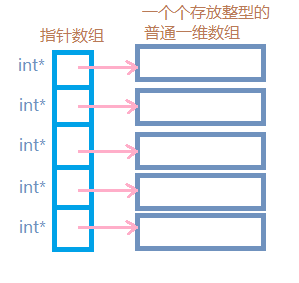# 动态创建二维数组的两种方法int **arr = NULL;
int row = 5;//用于表示行数
int col = 5;//用于表示列数
arr = new int*[row];//开辟一块内存来存放每一行的地址
for (int i = 0; i < row; i++)//分别为每一行开辟内存
arr[i] = new int[col];

int row = 6;
int col = 6;
vector<vector<int>> array(row);//创建一个有row行的二维数组arr
for (int i = 0; i < col; i++)
array[i].resize(col);//设置每一行的元素个数，即列数